Metrication.com      Introduction    Metric Units    Metric Conversions    Metrics in Drafting    Metrics in Engineering      Metric Drills      Metric Threads      Metric Fasteners    • Metric Gears     Metrics in Clothing    Metrics in the Home    Metric Products                and Information      Metric Converter Apps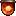FREE on selected platforms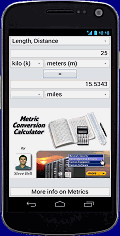For more, visit my main website: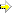Copyright © 2002-2014 Digital Design Ltd.Bookmark & Share: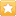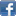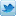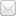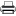## Metric Gears

Metric gears are defined by the module system, as follows: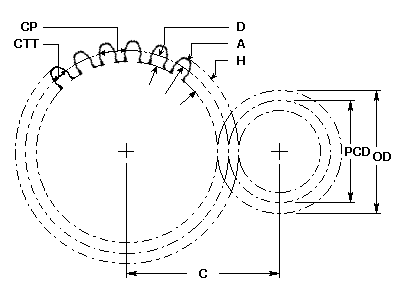###### Module
The length in mm of the pitch circle diameter per tooth.

MOD = PCD / N

```
```

###### Number of Teeth
The number of teeth on the gear.

N = PCD / MOD

```
```

###### Pitch Circle Diameter
The diameter of the pitch circle.

PCD = N x MOD

```
```

###### Outside Diameter
The outside diameter of the gear.

OD = (N + 2) x MOD

```
```

###### Center Distance
The distance between the axes of two gears in mesh.

C = PCD(g) + PCD(p)
2

```
```

###### Circular Pitch
The distance between adjacent teeth measured along the arc at the pitch circle diameter.

CP =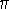x MOD

```
```

###### Circular Tooth Thickness
The width of a tooth measured along the arc at the pitch circle diameter.

CTT = CP / 2

```
```

The height of the tooth above the pitch circle diameter.

A = MOD

```
```

###### Dedendum
The depth of the tooth below the pitch circle diameter.

D = H - A

```
```

###### Whole Depth
The total depth of the space between adjacent teeth.

Finer than 1.25 MOD:
H = 2.4 x MOD

1.25 MOD and coarser:
H = 2.25 x MOD